Study S3 A Math Equations and Inequalities - Geniebook# Equations And Inequalities

• Relationship between the number of points of intersection and the nature of solutions of a pair of simultaneous equations
• Related conditions for a given line to
• intersect a given curve
• be a tangent to a given curve
• not intersect a given curve

The general solution of the equation $$ax^2 +bx + c =0$$  where $$a\neq 0$$,  are solved using the quadratic formula, $$x = {-b \pm \sqrt{b^2-4ac} \over 2a}$$  where $$x$$ is called the roots of the equation. We will be using this to understand the nature of the roots.

## Discriminant And Nature Of Roots

A discriminant, $$b^2-4ac$$, is a part of the quadratic formula which can be used to tell us the number of roots a quadratic equation has and nature refers to the type of roots that exist.

For the quadratic formula \begin{align} x = \frac{-b \pm \sqrt{b^2-4ac}}{2a} \end{align}

\begin{align} b^2 – 4ac \end{align}

Roots

Nature Of Roots

$$\gt0$$

\begin{align} x = {-b + \sqrt{b^2-4ac} \over 2a} \end{align}

\begin{align} x = {-b - \sqrt{b^2-4ac} \over 2a} \end{align}

2 real & distinct roots

$$=0$$

\begin{align} x &= \frac{-b \pm \sqrt{0}} {2a} \\ &=-\frac{b}{2a} \end{align}

2 real & repeated root

$$\lt0$$

\begin{align} x = {-b \pm \sqrt{\text{-ve}} \over 2a} \end{align}

Roots are

• Imaginary
• Complex
• No real roots

## Solving Linear Simultaneous Equations

Question 1:

Solve these simultaneous equations.

\begin{align} y &= 2x-1 \\ y &= -x+5 \end{align}

Solution:

You can solve this using the Substitution Method.

\begin{align} y &= 2x-1 & ---- \;(1) \\ y &= -x+5 & ----\;(2) \end{align}

Since, both equations equate to $$y$$ hence you can substitute the value of (1) in (2)

\begin{align} 2x - 1 &= -x+5\\ \implies\; 2x + x &= 1 + 5\\ \implies\qquad 3x &= 6 \\ \implies\qquad\;\; x &=2 \;\;\;\text{ is the root} \end{align}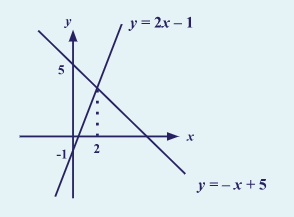## Solving Linear & Non–Linear Simultaneous Equation

Question 2:

Solve these simultaneous equations

\begin{align}​​ y &= x^2 +2x -5 \\ y &= -x+5 \end{align}

Solution:

You can solve this using the Substitution Method.

\begin{align}​​ y &= x^2 +2x -5 & ----\;(1)\\ y &= -x+5 & ----\; (2) \end{align}

Since both equations equate to $$y$$ hence you can substitute the value of (1) in (2)

\begin{align} -x+5 &= x^2+2x-5\\ \implies x^2 +2x -5 + x -5 &= 0\\ \implies\qquad\quad x^2 +3x -10 &= 0\\ \\ \end{align}

\begin{align} \therefore\quad x &= {-(3) \pm \sqrt{3^2-4(1)(-10)} \over 2(1)} \\ \implies x &= {-3 \pm \sqrt{49} \over 2} \\ \implies x &=2 \qquad \text{or} \qquad -5 \end{align}

Thus, the discriminant $$b^2 -4ac = 49$$ which is $$> 0$$ and hence there are two real roots, $$2$$ and $$–5$$.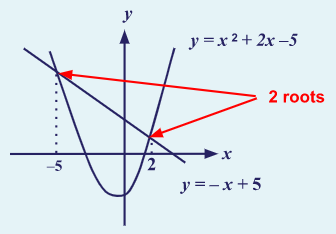Question 3:

Solve these simultaneous equations

\begin{align}​​ y &= x^2 -11x +30 \\ y &= -x +5 \end{align}

Solution:

You can solve this using the Substitution Method.

\begin{align}​​ y &= x^2 -11x +30 & ----\;(1)\\ y &= -x +5 & ----\;(2) \end{align}

Since both equations equate to $$y$$ hence you can substitute the value of (1) in (2)

\begin{align} x^2-11x+30 &= -x+5\\ \implies\;\; x^2 -11x +30 +x-5 &= 0\\ \implies\qquad\qquad x^2 -10x +25 &= 0 \end{align}

\begin{align}​​ \therefore\quad x &= {-(-10) \pm \sqrt{(-10)^2-4(1)(25)} \over 2(1)} \\ \implies x &= {10 \pm \sqrt{0} \over 2}\\ \implies x &=5 \end{align}

Thus, the discriminant is $$b^2 -4ac = 0$$ which is $$= 0$$ and hence there is one real distinct root, 5.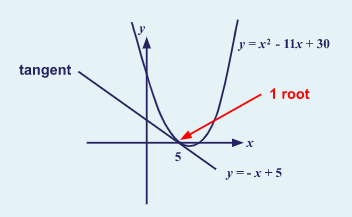Question 4:

Solve these simultaneous equations

\begin{align}​​ y &= x^2 -x +6 \\ y &= -x +5 \end{align}

Solution:

You can solve this using the Substitution Method.

\begin{align}​​ y &= x^2 -x +6 & ----\;(1)\\ y &= -x +5 & ----\;(2) \end{align}

Since both equations equate to $$y$$ hence you can substitute the value of (1) in (2).

\begin{align} x^2-x+6 &= -x+5\\ \implies x^2 -x +6 +x-5 &= 0\\ \implies\qquad\quad x^2 +0x+1 &= 0 \end{align}

\begin{align}​​ \therefore\quad\qquad x &= {-(0) \pm \sqrt{(0)^2-4(1)(1)} \over 2(1)}\\ \implies\qquad x &= {-(0) + \sqrt{-4} \over 2} \\ \implies\qquad x &= {-(0) - \sqrt{-4} \over 2} \end{align}

As the discriminant $$b^2 -4ac = -4$$  which is $$< 0$$ and hence there are no real distinct roots or no solution possible.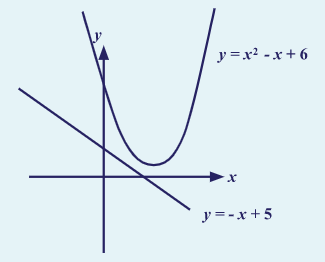## Related Conditions For Determining The Number Of Points Of Intersection Of A Line And A Curve

When two equations are given in the form

\begin{align}​​ y &=px^2 +qx +r \\ y &= ax +b \end{align}

You can solve them using \begin{align}​​ x = {-b \pm \sqrt{b^2-4ac} \over 2a} \end{align}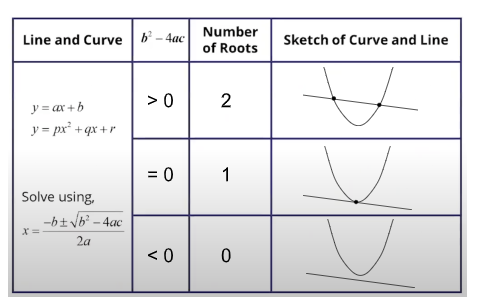Example 1:

Find the value of $$m$$ for which the line $$y = mx -3$$ is a tangent to the curve \begin{align}​​ y=x+\frac {1}{x} \end{align}.

Solution:

When we look at the question, it mentions a tangent to the curve $$y=x+\frac {1}{x}$$ which means the curve gets cut only at one point. It means that $$b^2 -4ac = 0$$ and there is only one real root.

\begin{align}​​ y &= mx -3 & ----\;(1)\\ y &=x+\frac {1}{x} & ----\;(2) \end{align}

\begin{align} \implies\qquad\qquad\quad\; mx-3 &= x+ \frac{1}{x}\\ \implies\qquad\qquad\quad\; mx -3 &= \frac{x^2+1}{x}\\ \implies\qquad\qquad\; mx^2-3x &= x^2 +1\\ \implies\; x^2+1 -mx^2 +3x &=0\\ \implies (1-m)x^2 +3x +1 &=0 \end{align}

Now we have  $$a=(1-m),\; b=3,\; c=1$$

We already know $$b^2 -4ac = 0$$

Hence,

\begin{align}​​ \implies 3^2 -4(1-m)(1)&=0\\ \implies\qquad\qquad\;\;\, 1-m &=\frac{9}{4} \\ \implies\qquad\qquad\qquad\;\, m &=1-\frac{9}{4} \\ \implies\qquad\qquad\qquad\;\, m &=-\frac{5}{4} \end{align}

Question 5:

Find the value of $$m$$ for which the line $$y = x+m$$ is a tangent to the curve $$y = 2x^2 -1$$.

Solution:

When we look at the question, it mentions a tangent to the curve $$y = 2x^2 -1$$, which means the curve gets cut only at one point. It means that $$b^2 -4ac = 0$$ and there is only one real root.

Equating both equations with each other we get,

\begin{align} x+m &=2x^2 -1\\ \implies\;\;\, 2x^2 -x-m-1 &= 0\\ \implies 2x^2 -x-(m+1 ) &= 0 \end{align}

Hence, we obtain $$a=2,\; b=-1 \;\text{and} \; c=-(m+1)$$

\begin{align} \therefore\qquad(-1)^2 -4(2)(-m-1)&=0\\ \implies\qquad\qquad 1-8(-m-1) &= 0\\ \implies\qquad\qquad\quad\;\; 1+8m +8 &= 0\\ \implies\qquad\qquad\qquad\quad\; 8m +9 &= 0\\ \implies\qquad\qquad\qquad\qquad\quad\;\; m &= -\frac{9}{8}\\ \implies\qquad\qquad\qquad\qquad\quad\;\ m &= -1\frac{1}{8} \end{align}

## Conclusion

In this article, we have observed the relationship between the number of points of intersection and nature of solutions of a pair of simultaneous equations as per the Secondary 3 Additional Mathematics syllabus in Singapore.

We have also studied the related conditions for a given line to

• intersect a given curve
• be a tangent to a given curve
• not intersect a given curve

Multiple examples and questions are also given to aid in understanding these concepts better. Keep learning! Keep improving!'

Continue Learning
Quadratic Functions in Real-World Context Equations and Inequalities
Logarithmic Functions Surds
Polynomials & Cubic Equations Partial Fraction
Exponential Functions Coordinate Geometry (Circles)
Linear Law Binomial TheoremPrimaryPrimary 1Primary 2Primary 3Primary 4Primary 5Primary 6SecondarySecondary 1Secondary 2Secondary 3PhysicsChemistryMathsE-MathsA-Maths
Equations and Inequalities
Logarithmic Functions
Surds
Polynomials & Cubic Equations
Partial Fraction
Exponential Functions
Coordinate Geometry (Circles)
Linear Law
Binomial TheoremSecondary 4